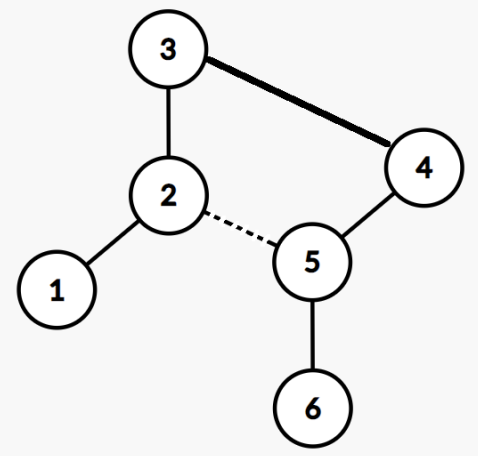시간 제한메모리 제한제출정답맞힌 사람정답 비율
3 초 1024 MB62240.000%

## 문제

A country has $N$ cities numbered from $1$ to $N$ and $N - 1$ bidirectional highways. It is possible to travel from any city to any other city using only the highways.

The distance between two cities $x$ and $y$ is defined as the number of highways required to travel from $x$ to $y$.

The governor has decided to demolish a highway and build another highway such that the largest distance between any two cities is maximized.

Find this maximum largest distance.

## 입력

The first line contains an integer, $N$, the number of cities.

In the next $N - 1$ lines, each line contains $2$ distinct integers $u$ and $v$, representing a highway connecting cities $u$ and $v$.

## 출력

Your program must print to standard output.

The output should contain a single integer on a single line, the new largest distance between any two cities.

## 제한

• $3 ≤ N ≤ 300\,000$
• $1 ≤ u, v ≤ N$

## 서브태스크

번호배점제한
15

$N ≤ 10$

210

$N ≤ 100$

315

$N ≤ 3000$

415

$N ≤ 300\,000$, there is at most one city with at least 3 highways connected to it.

555

-

## 예제 입력 1

4
1 2
1 3
3 4


## 예제 출력 1

3


It is impossible to increase the largest distance beyond 3.

This testcase is valid for all subtasks.

## 예제 입력 2

6
1 2
2 3
2 5
4 5
5 6


## 예제 출력 2

5


We may remove highway 2-5, and add the highway 3-4, so that the longest path becomes 1 − 2 − 3 − 4 − 5 − 6.This testcase is valid for subtasks 1, 2, 3 and 5.

## 채점 및 기타 정보

• 예제는 채점하지 않는다.